## Our Blog

### 2019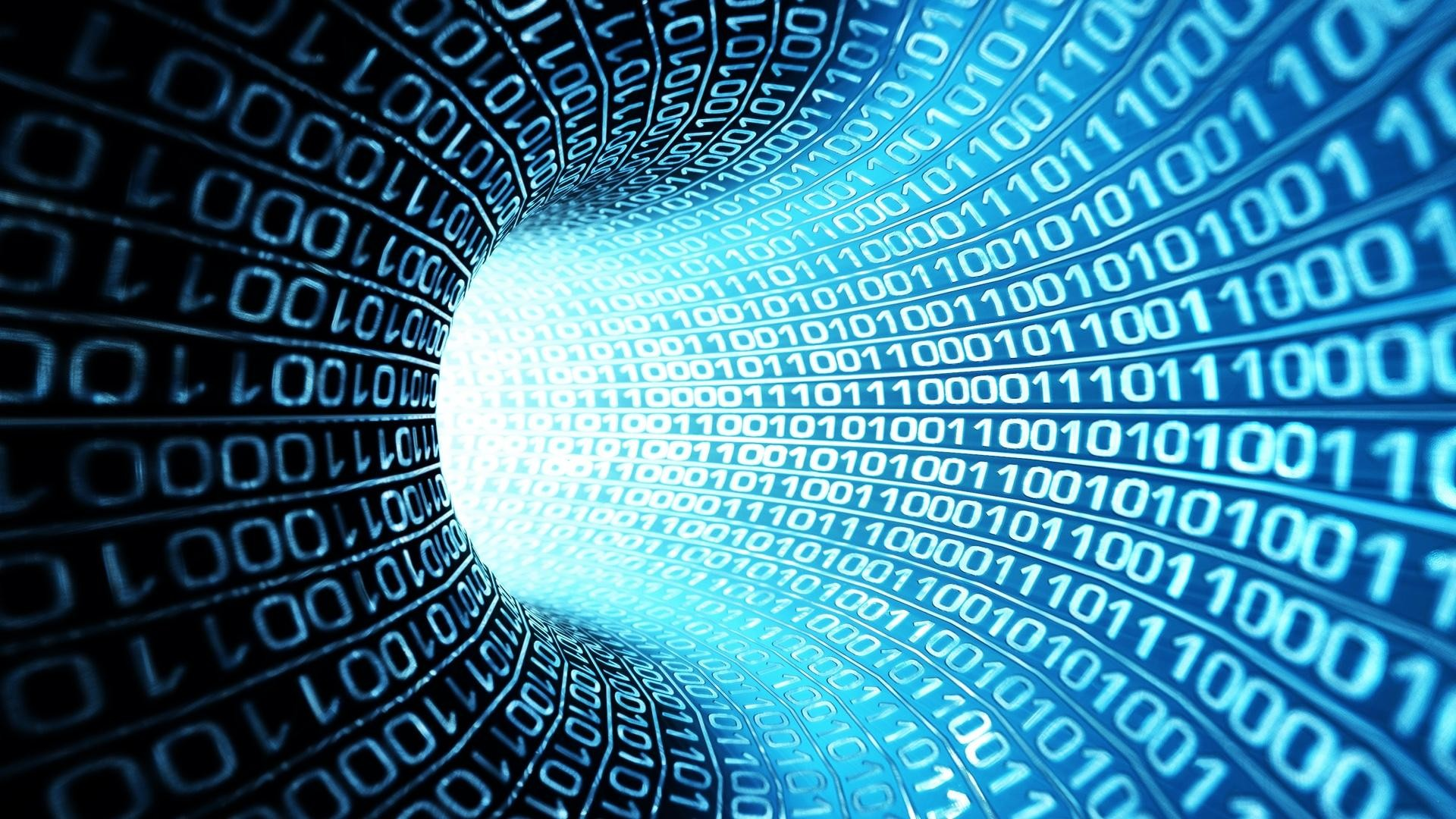### Number Systems

Have you ever wondered how computers are able to store data? Look no more! The answer is in the many existing number systems!### Cryptography

Do you enjoy solving things? Do you want to learn how to decode secret messages? Well, if you said “Yes!” to any of these questions, then this is the blog for you!### Finance

Show us the money!!!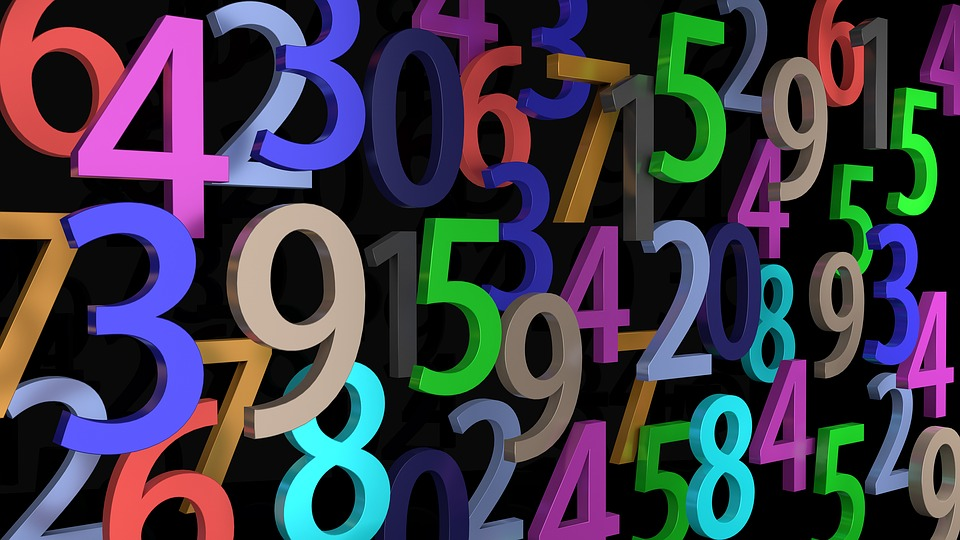### Number Theory

Number theory and its problems have stumped many mathematicians for centuries. Can you solve number theory’s greatest unsolved problems?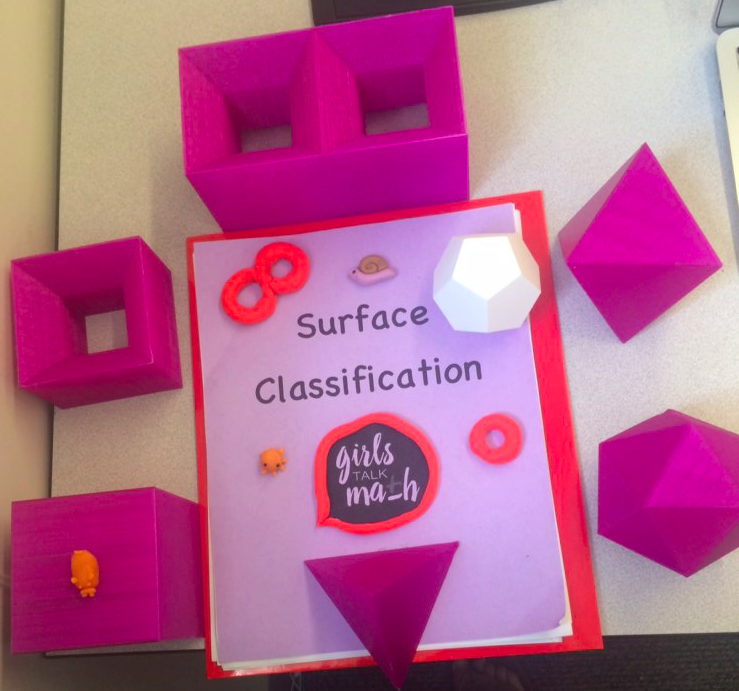### Surface Classification

What is a surface? Is there such a thing as a one-sided figure? What dimension is time in? In Surface Classification, we learned the answer to these questions and more.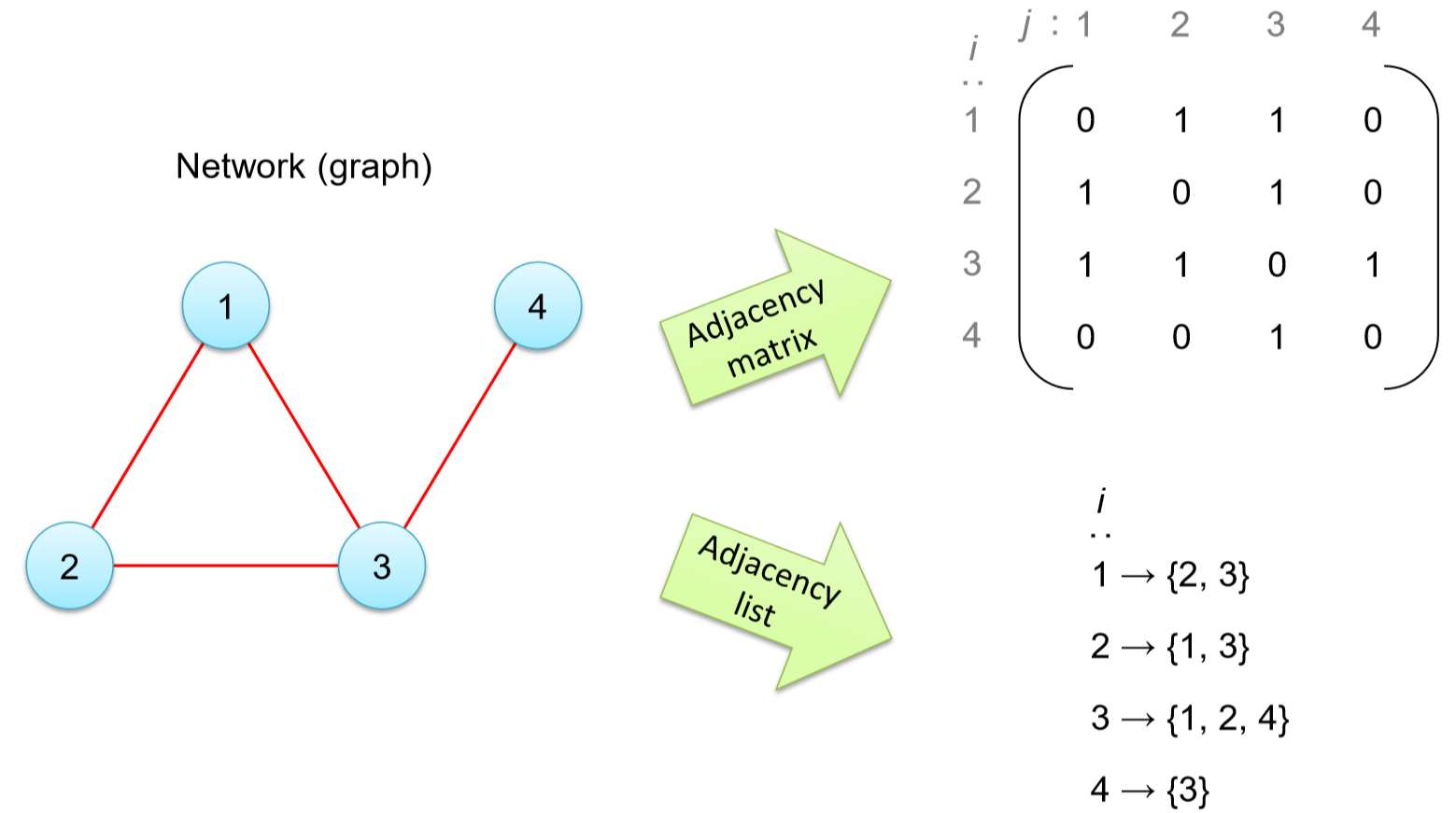### Network Models, Graph Theory and Algorithms

Graph theory is the study of mathematical structures called networks. Network science is a field that applies the mathematical results of graph theory to study network models of real world problems using things like Python and computer programming.### Quantum Mechanics

Quantum mechanics can get weird to say the very least, albeit very interesting. These past two weeks, we studied concepts including but not limited to light waves, polarization, and quantum entanglement (and socks).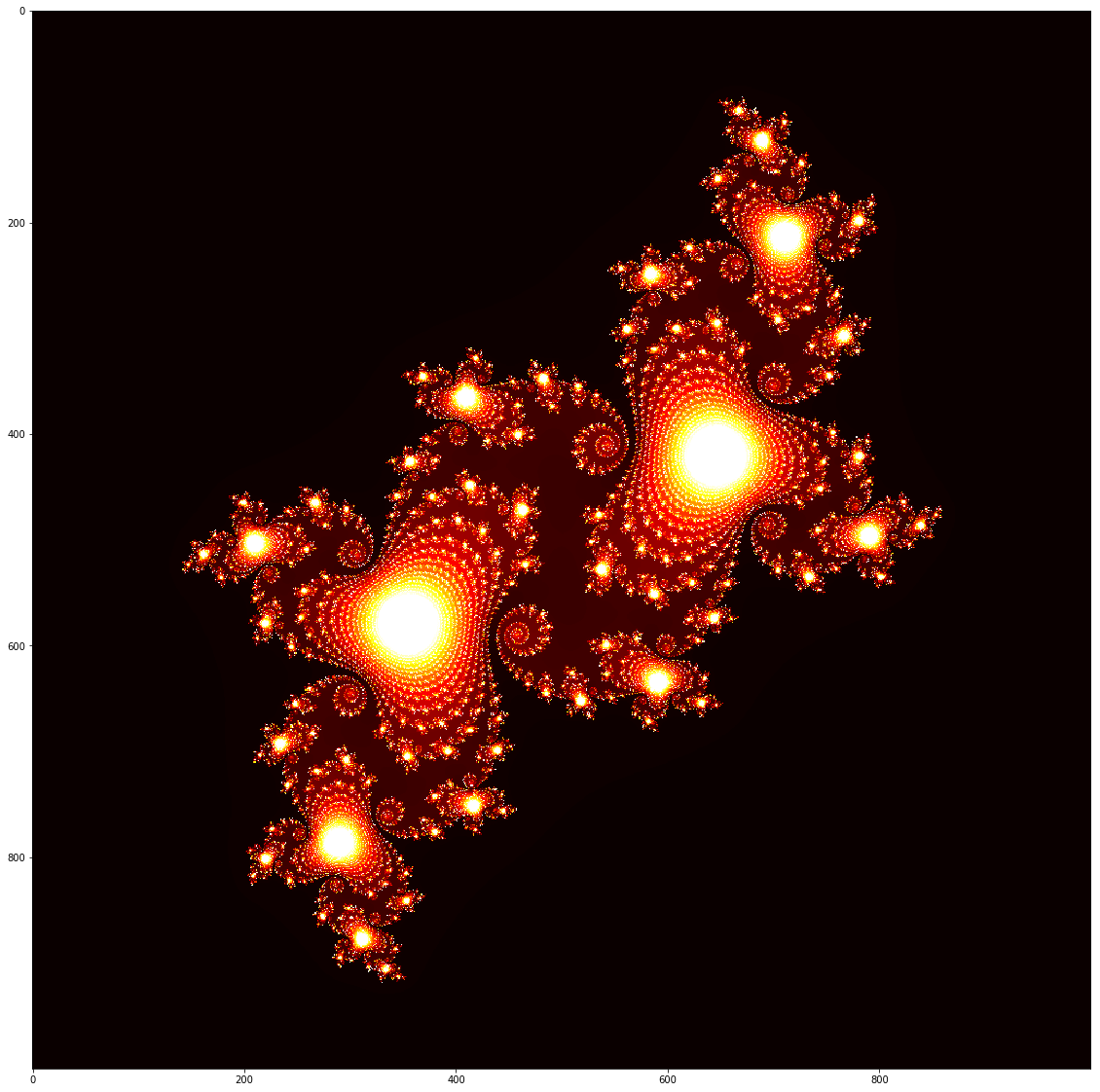### Patterns and Fractals

Learn about how patterns can forms in nature and how the behavior of iterated functions can be classified as fractals.